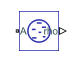# Liquid Properties Sensor (IL)

Measure density, bulk modulus, and fraction of entrained air in isothermal liquid network

Since R2020a

•Libraries:
Simscape / Foundation Library / Isothermal Liquid / Sensors

## Description

The Liquid Properties Sensor (IL) block represents an ideal sensor that lets you measure mixture density, bulk modulus, and fraction of entrained air in an isothermal liquid network. There is no mass flow through the sensor.

The block measures these properties at the node connected to port A. All measurements are taken with respect to absolute zero.

## Ports

### Output

expand all

Physical signal output port for measuring the density of isothermal liquid mixture.

#### Dependencies

This port is visible if you set the Density parameter to `On`.

Physical signal output port for measuring the bulk modulus of isothermal liquid mixture.

#### Dependencies

This port is visible if you set the Isothermal bulk modulus parameter to `On`.

Physical signal output port for measuring the fraction of entrained (undissloved) air, (entrained air)/(entrained air + dissolved air). Value of 1 means no air is dissolved. Value of 0 means all air is dissolved.

#### Dependencies

This port is visible if you set the Fraction of air that is entrained parameter to `On`.

### Conserving

expand all

Isothermal liquid conserving port. Connect this port to the node in the isothermal liquid network where you want to measure the liquid properties. All measurements are taken with respect to absolute zero.

## Parameters

expand all

Setting this parameter to `On` exposes output port rho, which lets you measure fluid density.

Setting this parameter to `On` exposes output port beta, which lets you measure bulk modulus.

Setting this parameter to `On` exposes output port theta, which lets you measure the fraction of undissolved air.

## Version History

Introduced in R2020a